Single-phase transformer

HOME / Applications / Single-phase transformer
WEBINAR
Simultaneous Wireless Information and Power Transfer
Thursday, January 27, 2022
Time
SESSION 1
SESSION 2
CET (GMT +1)
03:00 PM
08:00 PM
EST (GMT -4)
09:00 AM
02:00 PM
Used Tools:Single-phase transformer

In this application note, EMS is used to study a single-phase transformer. AC Magnetic module of EMS coupled to thermal solver both are utilized to compute and generate magnetic fields, electromagnetic losses including coreloss, excess loss, hysteresis loss, eddy loss, and temperature evolution of the studied transformer.

SOLIDWORKS model of a Single-phase transformer

The SOLIDWORKS model of a single-phase transformer consists of a core, an inner coil and an outer coil, as shown in Figure 1. The transformer core is made of M27.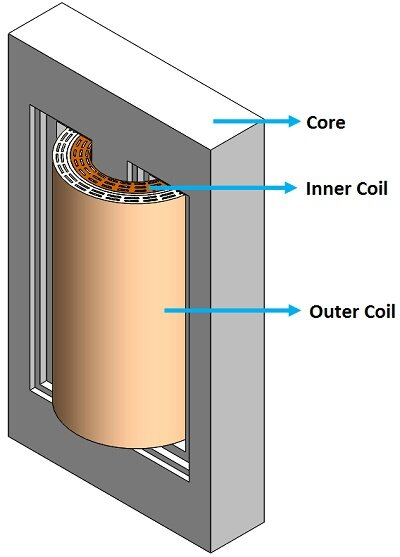Figure 1 - 3D model of a single phase transformer

Coupling to Thermal Analysis

Thermal analysis calculates the temperature distribution in a body due to conduction in the solids. A convection boundary condition is allowed to define what happens to the heat flux in the extremities of the model.

In EMS, Thermal analysis automatically follows an electromagnetic analysis so that the heat sources in the model are automatically pre-computed.

Material

The simulated model is composed of a laminated steel core, an inner coil, an outer coil and Air. The materials properties are summarized in Table 1. The coreloss PB curve of the core material is shown in Figure 2.

Table 1 - Materials properties

 Component Material Relative permeability Electrical Conductivity (S/m) Thermal Conductivity (W*m-1  * k-1) Core laminated steel (M27 at 0.36mm; Mass density: 7650 kg/m^3) Nonlinear 2.32558 e+006 43 Inner Coil/ Outer Coil Copper 0.99991 5.7e+007 401 Coils Air, Inner Air, Outer Air Air 1 0 0.024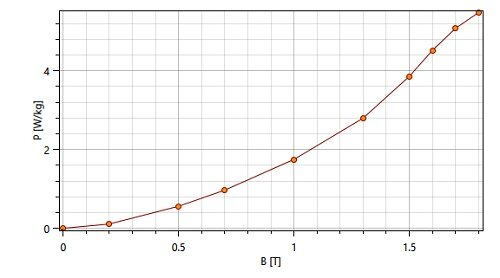Figure 2 - Core loss curve

Primary/ secondary windings - Open circuit case

In this simulation, the inner and outer coils are modeled as wound coils. Primary winding is current driven coil with an input current of  10*300 A-turns.The secondary side of the transformer is made of 600 turns open-circuit winding. The operating frequency is 60Hz.

Thermal Inputs

Convection is the heat transfer mode in which heat transfers between a solid face and an adjacent moving fluid (or gas). In EMS, all air bodies (Outer Air, Inner Air and Coils Air) are applied a convection boundary condition.
Convection coefficient was set to be   10 W/ (m2 *k), and the Bulk ambient temperature was set to be 300 Kelvin.

Mesh

To achieve a good accuracy without increasing the total number of mesh elements, it is recommended to apply a mesh control to the regions where a large variation is expected. Figure 3 shows the resulting mesh.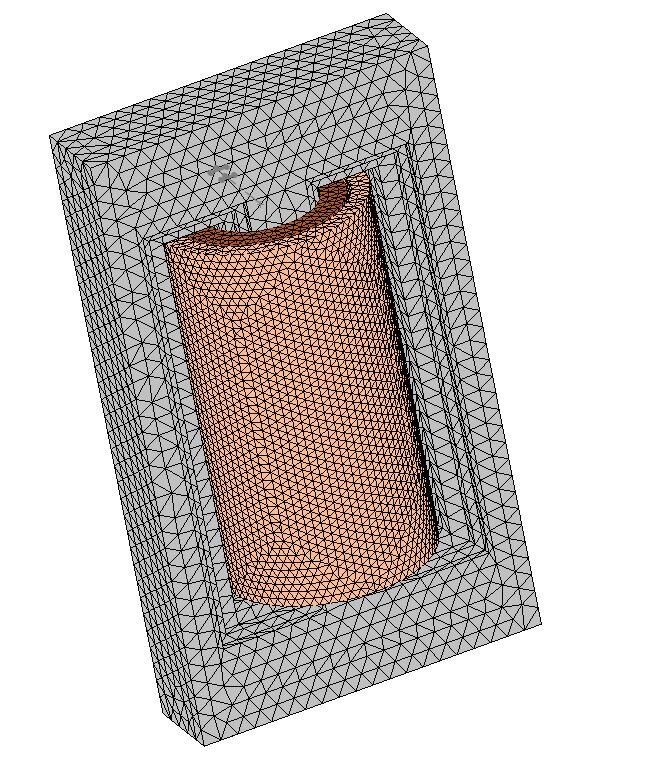Figure 3 - Meshed model

Simulation and results - Open circuit condition

EMS enables to compute and generate several output data, including magnetic fields, EM losses, currents, voltages, inductance and resistance matrices, etc, which allow to design and optimize different types of transformers. Figure 4 shows the magnetic flux density of the transformer core. The maximum magnetic fields are located along the middle leg of the transformer. It reaches 2.1T. The fluxes in both sides legs are ranging from 1T to 1.7T. Cross section view of the magnetic field vector plot is demonstrated in Figure 5.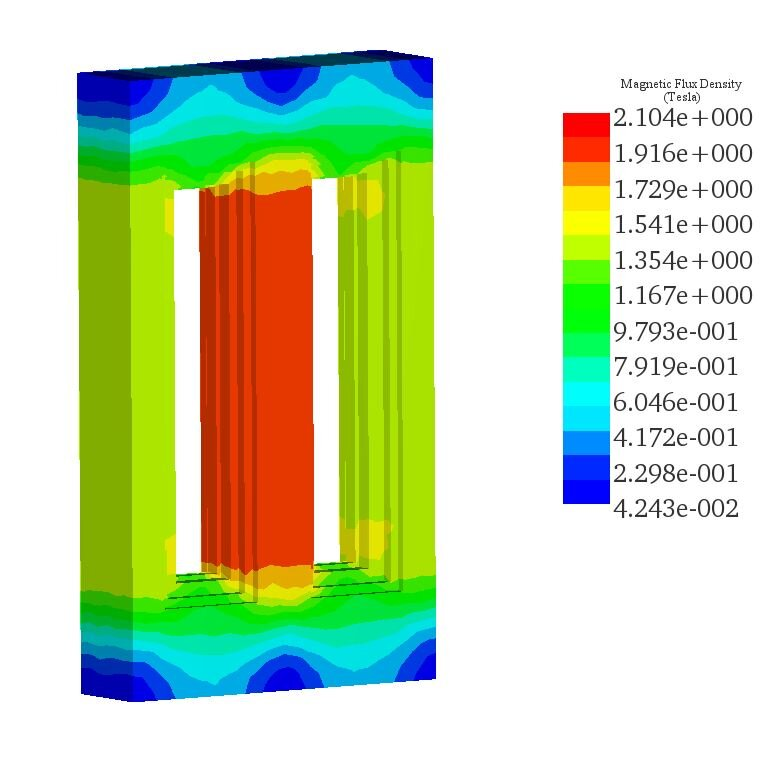Figure 4 - Magnetic flux density in the transformer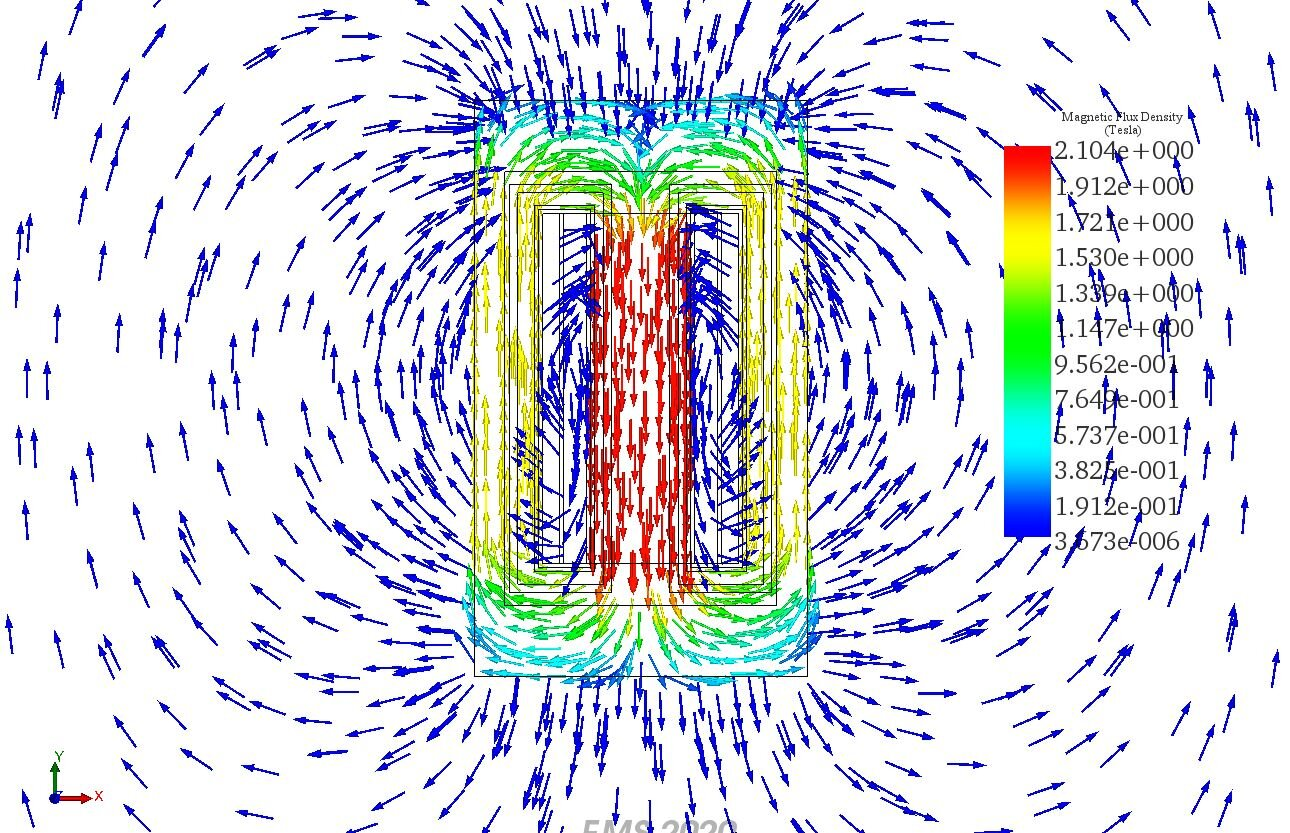Figure 5 - Vector plot of the magnetic flux

Multiple cross sections plot of the H field is shown in Figure 6. Maximum H field is around 3.24e+3 Amp/m reached in the transformer middle leg.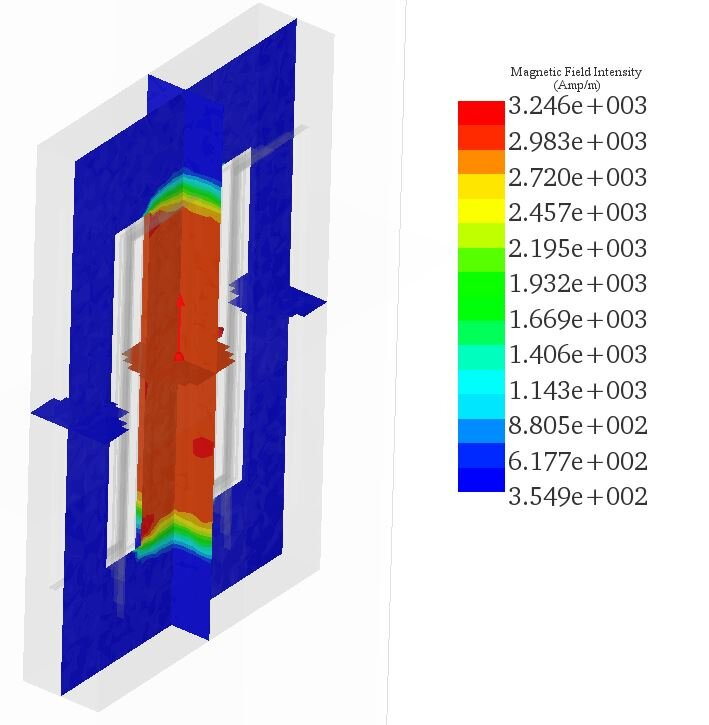Figure 6 - H field plot

Coreloss results density is plotted in Figure 7 while the lumped results of the coreloss is around 8.9kW. It includes mainly hysteresis and eddy losses. Both iron and winding losses are sources of heat in the transformer due to the Joule effect. Figure 8 shows the temperature results generated in the single phase transformer. The maximum steady state temperature is aboutwhich is mainly caused by the coreloss. Cross section view of the transformer illustrates the temperature inside the transformer core. The highest temperature is located at the middle leg of the transformer.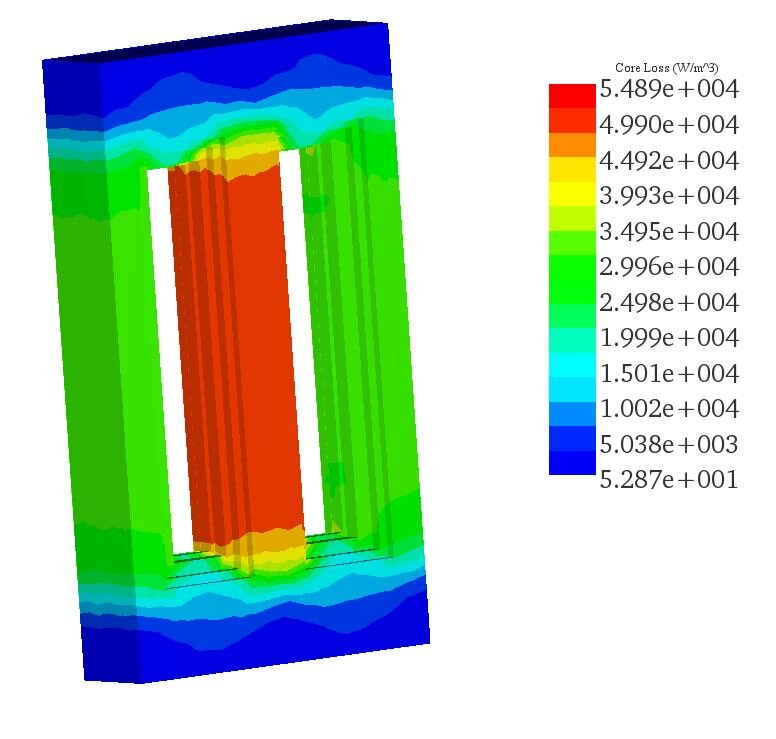Figure 7 - Coreloss density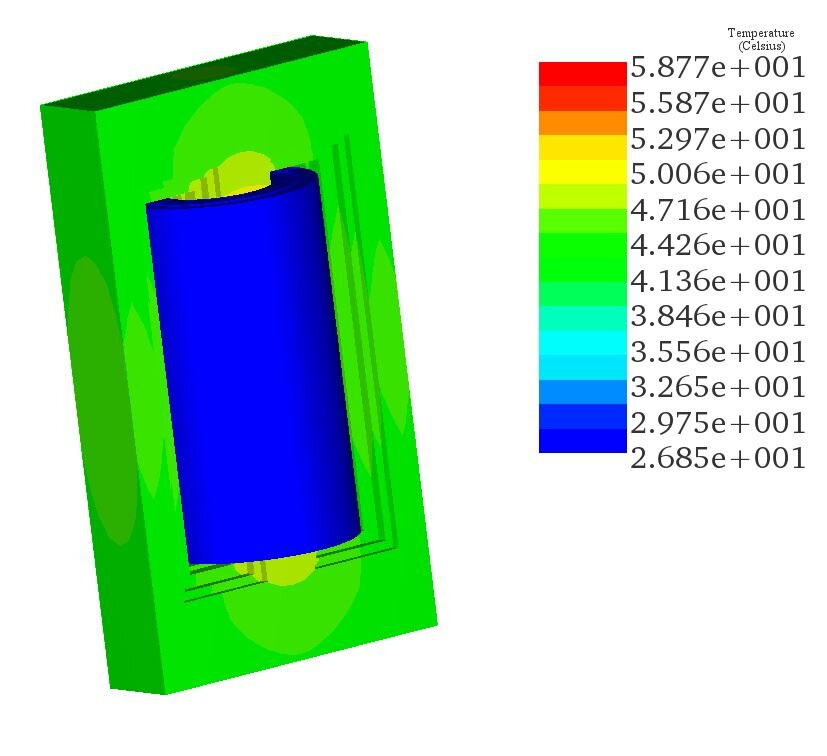Figure 7 - Temperature results of the transformer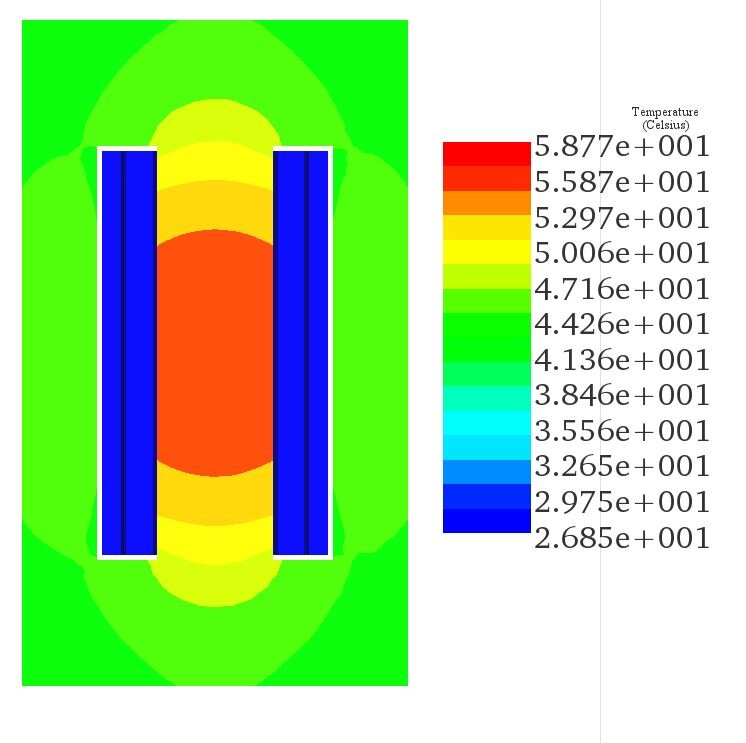Figure 7 - Cross section view of the temperature results

Conclusion

Using EMS, the AC Magnetic study coupled to thermal analysis helped to study and compute different results related to a single phase transformer. Magnetic fields, EM losses, winding parameters, temperature are calculated and generated under open-circuit condition. Moreover, EMS allows to simulate transformers under different conditions such as short circuit, with loads (resistive, capacitive and inductive loads) and multiple connections types, etc.

Videos